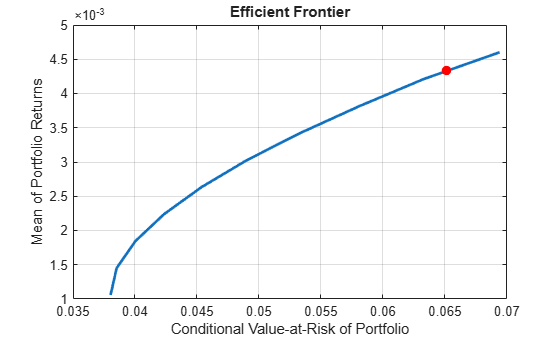# Compute Maximum Reward-to-Risk Ratio for CVaR Portfolio

Create a `PortfolioCVaR` object and incorporate a list of assets from `CAPMUniverse.mat`. Use `simulateNormalScenariosByData` to simulate the scenarios for each of the assets. These portfolio constraints require fully invested long-only portfolios (nonnegative weights that must sum to `1`).

```rng(1) % Set the seed for reproducibility. load CAPMuniverse p = PortfolioCVaR('AssetList',Assets(1:12)); p = simulateNormalScenariosByData(p, Data(:,1:12), 20000 ,'missingdata',true); p = setProbabilityLevel(p, 0.95); p = setDefaultConstraints(p); disp(p)```
``` PortfolioCVaR with properties: BuyCost: [] SellCost: [] RiskFreeRate: [] ProbabilityLevel: 0.9500 Turnover: [] BuyTurnover: [] SellTurnover: [] NumScenarios: 20000 Name: [] NumAssets: 12 AssetList: {1x12 cell} InitPort: [] AInequality: [] bInequality: [] AEquality: [] bEquality: [] LowerBound: [12x1 double] UpperBound: [] LowerBudget: 1 UpperBudget: 1 GroupMatrix: [] LowerGroup: [] UpperGroup: [] GroupA: [] GroupB: [] LowerRatio: [] UpperRatio: [] MinNumAssets: [] MaxNumAssets: [] BoundType: [12x1 categorical] ```

To obtain the portfolio that maximizes the reward-to-risk ratio (which is equivalent to the Sharpe ratio for mean-variance portfolios), search on the efficient frontier iteratively for the porfolio that minimizes the negative of the reward-to-risk ratio:

`$-\text{\hspace{0.17em}}\frac{\mathrm{portfolio}\text{\hspace{0.17em}}\mathrm{return}-\mathrm{risk}\text{\hspace{0.17em}}\mathrm{free}\text{\hspace{0.17em}}\mathrm{rate}}{\mathrm{portfolio}\text{\hspace{0.17em}}\mathrm{CVaR}}.$`

To do so, use the `sratio` function, defined in the Local Functions section, to return the negative reward-to-risk ratio for a target return. Then, pass this function to `fminbnd`. `fminbnd` iterates through the possible return values and evaluates their associated reward-to-risk ratio. `fminbnd` returns the optimal return for which the maximum reward-to-risk ratio is achieved (or that minimizes the negative of the reward-to-risk ratio).

```% Obtain the minimum and maximum returns of the portfolio. pwgtLimits = estimateFrontierLimits(p); retLimits = estimatePortReturn(p,pwgtLimits); minret = retLimits(1); maxret = retLimits(2); % Search on the frontier iteratively. Find the return that minimizes the % negative of the reward-to-risk ratio. fhandle = @(ret) iterative_local_obj(ret,p); options = optimset('Display', 'off', 'TolX', 1.0e-8); optret = fminbnd(fhandle, minret, maxret, options); % Obtain the portfolio weights associated with the return that achieves % the maximum reward-to-risk ratio. pwgt = estimateFrontierByReturn(p,optret)```
```pwgt = 12×1 0.0885 0 0 0 0 0.9115 0 0 0 0 ⋮ ```

Use `plotFrontier` to plot the efficient frontier and `estimatePortRisk` to estimate the maximum reward-to-risk ratio portfolio.

```plotFrontier(p); hold on % Compute the risk level for the maximum reward-to-risk ratio portfolio. optrsk = estimatePortRisk(p,pwgt); scatter(optrsk,optret,50,'red','filled') hold off```### Local Functions

This local function that computes the negative of the reward-to-risk ratio for a target return level.

```function sratio = iterative_local_obj(ret, obj) % Set the objective function to the negative of the reward-to-risk ratio. risk = estimatePortRisk(obj,estimateFrontierByReturn(obj,ret)); if ~isempty(obj.RiskFreeRate) sratio = -(ret - obj.RiskFreeRate)/risk; else sratio = -ret/risk; end end```